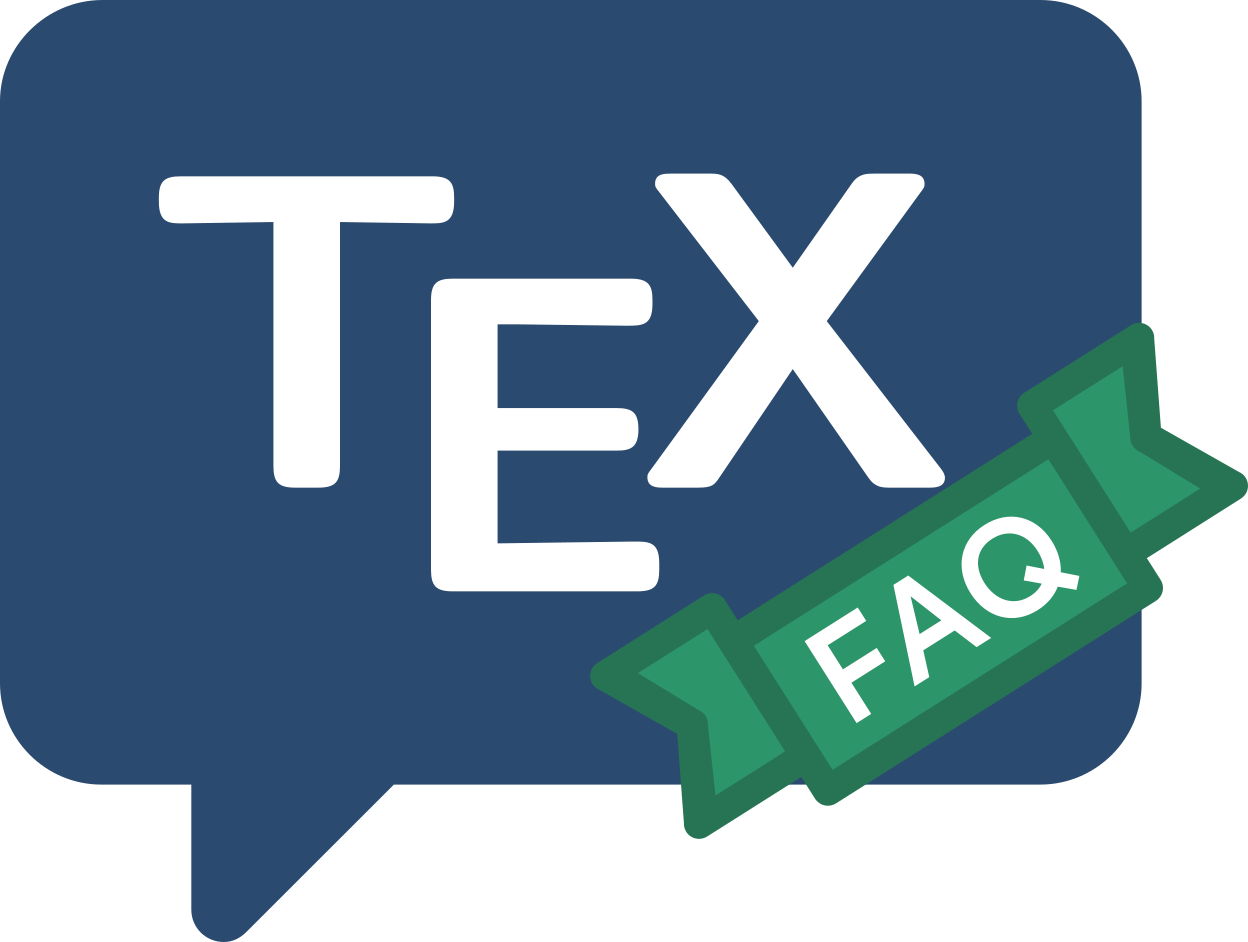Frequently Asked Question List for TeX

# Am I using pdfTeX, XeTeX or LuaTeX?

You often need to know what “engine” your macros are running on (the engine is the TeX-derivative or TeX-alike processor that typesets your document). The reason that you need to know is that the set of functions available in each engine is different. Thus, for TeX macros to run on any engine, they need to “know” what they can and cannot do, which depends on the engine in use. Getting the right answer is surprisingly tricky.

There is now a comprehensive package that answers the question for you.

This provides TeX conditionals to allow testing for various engine and output modes.

`\ifpdf` (true if outputting PDF) `\ifxetex` (true if using xetex), `\ifluatex` (true for luatex and luahbtex), `\ifluahbtex` (true if the Lua Harfbuzz library is available),`\ifptex` (true for Japanese pTeX variants) etc.

For historical reasons these commands are also provided with mixed case names, `\ifXeTeX` for example.

These TeX commands may also be used within the LaTeX conditional framework, as (for example):

``````\ifthenelse{\boolean{pdf}}{<if pdf>}{<if not pdf>}
``````

One special conditional is `\iftutex` which is true for Unicode TeX engines (LuaTeX and XeTeX) and allows a simple switch to select Unicode font packages such as

``````\iftutex
\usepackage{fontspec}
\setmainfont{TeX Gyre Termes}
\else
\usepackage{times}
\fi
``````

The package also provides commands such as `\RequireXeTeX` corresponding to each of the engine tests. these create an error and stop the job if the code is not running under the correct engine.

FAQ ID: Q-whatengine
Tags: macrosluatexxetex